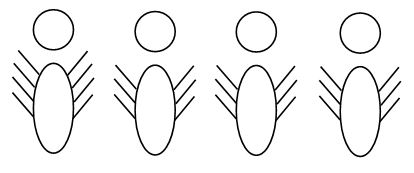# Maths Logic Problems

A favourite of examiners, particularly of those setting questions for independent schools, is the mathematical logic puzzle. It'd be impossible to address all the possible permutations here but we are looking at a question such as:

If there are eight-legged spiders and six-legged ants in a jar and the total number of legs is 28, how many of each creature are there?

Immediately it is clear that it's a maths question but the process required to solve it isn't. Children approaching these questions have to stop, think and visualise to give them a shot at finding the right way to solve it. There are several ways...Here I've sketched one spider and three ants, purely because it gives us an answer that is ROUGHLY what we are after. It doesn't matter that it's not what we need precisely - a ballpark figure gives us the starting point needed. We can solve this through trial and error.

Having sketched it out (I could, for speed purposes, have simply written 8, 6, 6, 6) I can count up the legs and see whether it equals 28. As it doesn't, but I'm two short, I can change an ant to a spider which will then give the required number. The answer could therefore be two ants and two spiders.

An alternative way to solve it could be to count up in eights or sixes.

8 + 8 + 8 = 24
+ 4 (not 6 or 8) = 28 so there cannot be an answer when you have three spiders

6 + 6 + 6 + 6 = 24
+ 4 (not 6 or 8) = 28 so again, we cannot solve the problem with four ants.

You might strike lucky doing it this way but the technique is to count in sixes until the remainder is in the eight times table, or vice versa.

6 + 6 = 12

28 - 12 = 16 (16 is in the eight times table so we have (16 ÷ 8) two spiders to add to the two ants.